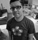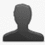This project is archived and is in readonly mode.

✓resolved## Add support for rgba() and hsla()

Reported by Oskar Krawczyk | December 15th, 2008 @ 12:17 AM | in 2.0 (closed)

This would come in handy and with any luck should be implemented inside 1.2.2 since this would mostly be used within AIR apps.

For specs please refer to the CSS3 Color Module: http://www.w3.org/TR/2003/CR-css...

Ie:

``````
element {
color: rgba(255, 255, 255, 0.5);
}
``````

### Comments and changes to this ticket

•#### nak5iveFebruary 27th, 2009 @ 05:53 AM

• Tag changed from 122 to 122, color, hsl, rgb

these should help get started...

``````
function rgbToHsl(r, g, b) {
r /= 255, g /= 255, b /= 255;
var max = Math.max(r, g, b), min = Math.min(r, g, b);
var h, s, l = (max + min) / 2;

if (max == min)
h = s = 0;
else {
var d = max - min;
s = l > 0.5 ? d / (2 - max - min) : d / (max + min);
switch (max) {
case r :
h = (g - b) / d + (g < b ? 6 : 0);
break;
case g :
h = (b - r) / d + 2;
break;
case b :
h = (r - g) / d + 4;
break;
}
h /= 6;
}

return [h, s, l];
}

function hslToRgb(h, s, l) {
var r, g, b;

if (s == 0)
r = g = b = 1;
else {
function hue2rgb(p, q, t) {
if (t < 0)
t += 1;
if (t > 1)
t -= 1;
if (t < 1/6)
return p + (q - p) * 6 * t;
if (t < 1/2)
return q;
if (t < 2/3)
return p + (q - p) * (2/3 - t) * 6;
return p;
};

var q = l < 0.5 ? l * (l + s) : l + s - l * s;
var p = 2 * l - q;

r = hue2rgb(p, q, h + 1/3);
g = hue2rgb(p, q, h);
b = hue2rgb(p, q, h - 1/3);
}

return [(r * 255).round(), (g * 255).round(), (b * 255).round()];
}
``````
•#### Christoph PojerJune 4th, 2009 @ 10:45 PM

• State changed from “new” to “resolved”

This will be in MooTools 2.0, until then you can use mootools-color: http://github.com/kamicane/mootools-color/tree/master

•#### Oskar KrawczykJune 4th, 2009 @ 10:53 PM

I thought so. Also, I did gave mootools-color a try, and whatever I do, it doesn't seem to take the forth param in consideration.